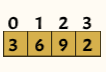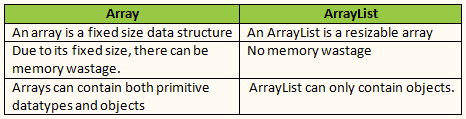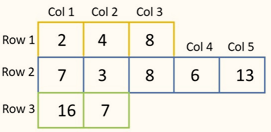# Top Java Array Interview Questions & Answers for Placement

1. What is an Array in Java? What are the types of Arrays?
2. What are the Advantages and Disadvantages of an Array?
3. What is the default value of the Array?
4. What is an Array of Objects?
5. How to create an Array of Objects in Java?
6. What is an ArrayList in Java? What is the difference between an Array and ArrayList?
7. What are the Advantages of a LinkedList over an Arraylist? In which scenarios do we use LinkedList over ArrayList?
8. What is a Jagged Array in Java? What is the difference between Jagged Array and Multidimensional Array?

## 1. What is an Array in Java? What are the types of arrays?

An array is a data structure that can hold multiple values which are of the same type. The size of an array is defined when it is created and it cannot be changed meaning the size of the array cannot be increased or decreased once it is declared. Each value in an array can be accessed using an index that starts from 0 (zero).This is an array of 4 elements. You can see all the elements are integers and homogeneous. There is index of the array which always starts from zero and goes up to n-1 elements.
Let’s see an example of 1D  Array in a Java program

#### Example:

public class Demo {
public static void main(String[] args) {
int[] myNum;   // declare an array of type int
myNum = new int;   // an array is created with size 10
myNum = 11; // initialize the array
myNum = 8;
myNum = 7;
System.out.println("First Element: " + myNum);
}
}

There are two types of arrays in Java, there are one-dimensional arrays which we have just discussed and there are multi-dimensional arrays.

A multi-dimensional array is an array that has more than one dimension. It is an array of arrays, an array that has multiple levels or dimensions.
Multi-dimensional arrays can be 2D or 3D arrays.

A 2D array is an array of arrays where each element inside the array is a 1D array.

2D array is a matrix and is well-suited for spreadsheet-type data. For example, we can have a 2D array for 3 students to store marks for 4 subjects.

int[][] a = new int;//3 row and 4 columnA 3D array is an array where each element inside the array is a 2D array.

For example, we can have a 3D array for 3 students to store scores on 4 subjects for 4 different term exams.

int[][] a = new int{4};

Here, the first dimension indicates the number of Tables/Arrays, the second dimension indicates the number of Rows and the third dimension indicates the number of Columns.

## What are the Advantages and Disadvantages of an Array?

Advantages of an array:

- An array can store multiple values in a single variable.
- It is easy to access any element randomly using the index of array
- We can also loop through the array using the index to access the elements of an array quickly.
- It is easy to manipulate and store large data in an array

Disadvantages of an array:

- The size of the array cannot be changed.
- Since, the size of the array is fixed, there is a chance of memory wastage.
- Only items or objects of similar type can be store in an array.
- To modify elements of an array you need to traverse throughout the array which increases the time complexity.

## What is the default value of the Array?

When an array is created and the array is not initialized, then the compiler would assign them their default values, which are different for different types of array.

- For a Boolean array the default value is false.
- Integer array, is 0.
- Double array, is 0.0.
- Reference types, is null.

For example, the following java program with an integer array,

#### Example:

class Demo {
public static void main(String[] args) {
int[] myNum = new int; // an array is created but not initialized
System.out.println("First Element is " + myNum);
}
}

Output: First Element is 0

## What is an Array of Objects?

In Java, arrays can hold primitive values such as int, double, etc and reference values which points to objects. Just as the array that hold integer values is known as an integer array, the array which holds objects is known as an array of objects. Also note that the array can only hold the references to the objects and not the objects themselves.

## How to create an Array of Objects in Java?

To create an array of objects in Java, we use the following syntax:

Object[] objectArrayName = {list of objects};

The following program is an example of how to create an array of objects in Java.

#### Example:

class Main{
public static void main(String args[]){
Student[] obj = new Student ; //create array of student object
obj = new Student(1,"Ramesh"); //create & initialize actual Student
//  objects using constructor
obj = new Student(2,"Ganesh");
//display the Student object detail
System.out.println("Student Object 1:");
obj.showDetail();
System.out.println("Student Object 2:");
obj.showDetail();
}
}
//Student class with sId and name as attributes
class Student{
int stId;
String name;
//Student class constructor
Student(int id, String n){
sId = id;
name = n;
}
public void showDetail(){
System.out.print("StudentId = "+ studentId + "  " + " Student Name = "+name);
System.out.println();
}
}

Output
Student Object 1:
Id=1 Name = Ramesh
Student Object 2:
Id=2 Name = Ganesh

## What is an ArrayList in Java? What is the difference between an Array and ArrayList?

An ArrayList is a class that helps to create resizable arrays. It is also called a dynamic array. Unlike arrays, ArrayList  can automatically adjust their sizes when we add or remove elements from them.

To create an ArrayList, we need to import the “ java.util.ArrayList “ package first and we can create an integer ArrayList as follows:

ArrayListmyArrayList= new ArrayList<>();

As we can see, the wrapper class Integer is used instead of primitive int, this is because ArrayList can only work with wrapper classes.

#### Example:

import java.util.ArrayList;
public class Main {
public static void main(String[] args) {
ArrayList animals = new ArrayList();
System.out.println(animals.get(0)); // get() method is used
// to access an element
// in the ArrayList
}
}## What are the advantages of a Linked List over an Array? In which scenarios do we use Linked List over ArrayList?

In Java, a Linked List is a class just like an ArrayList which can help create a data structure that can contain many objects. A Linked List also has special methods such as add(), remove(), get() etc, just like an ArrayList.

But unlike an ArrayList that internally uses a dynamic array to store the elements., LinkedList uses a doubly linked list to store the elements.

Advantages of a Linked List over an Arraylist:

LinkedList is much faster as compare to ArrayList where manipulation like addition/deletion operations is frequent.

LinkedList uses a doubly linked list so the list elements can easily be inserted and removed without reorganization of the list because the elements do not require contiguous memory. In case of ArrayList, it uses an array internally. If any element is removed from the array, all the other elements are shifted in memory.

Linked List is used over ArrayList in the following scenarios:
LinkedList should be used where modifications rate is more than the read scenarios. And, when the rate of read scenarios is more than the addition or removal rate, then ArrayList should be used.

LinkedList maintains two links i.e next and previous links to store the address of the previous and the next nodes. So, memory overhead in the LinkedList is more as compared to the ArrayList where no such links are required.

## What is a Jagged Array in Java? What is the difference between jagged array and multidimensional array?

A Jagged array is an array of arrays or a multi-dimensional array where each inner array can be of different size, which means there can be variable number of columns in each row. And each of the member array occupy their own blocks in memory.

Below is an example of a 2D jagged array,

int[] [] jaggedArray = { new int [] { 2, 4, 8 },
new int [] { 7, 3, 8, 6, 13 },
new int [] { 16, 7 }
};

For exampleWhereas in a 2D multi-dimensional array, the number of columns is the same in each row which is usually represented in the form of a matrix. For example,

int[] [] myArray = new int ;  // 2D array having 4 rows and 4 columns